3rd of September, 2008

1. Solve the following system of equations in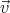: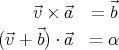2. Calculate the divergence and curl of the vector fields below (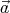and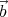are constant vectors). Which of these vector fields could be an electrostatic field?
1.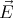(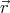) = (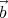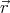)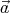2.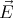(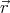) =3.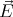(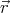) = r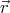4.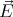(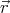) =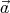× (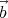×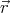)
5.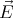(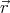) = (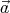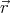)(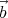×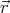)
6.(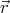) =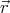× (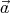×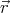)
3. A static charge distribution produces a radial electric field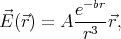where A and b are constants, and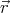is the position vector.

1. What is the charge density ρ(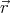)?
2. What is the total charge?
4. Consider a uniformly charged solid sphere of radius R and charge Q.
1. Sketch the electric field and potential as a function of the distance from the centre of the sphere.
2. What is the total electrostatic energy stored in the sphere?
5. The little green tin-mining men found a perfectly spherical planetoid, made of solid tin. They decided to conduct a mining operation on it. They began by extracting an equatorial section of the planetoid, essentially cutting it in half (see fig. 1). However, the mine roof support jacks started to fail, breaking under the weight of the half-spheres! Stronger jacks must be used to replace them.

The little green mining engineer was assigned the task to find the force necessary to keep the two halves of the planetoid apart. Help him with the calculations!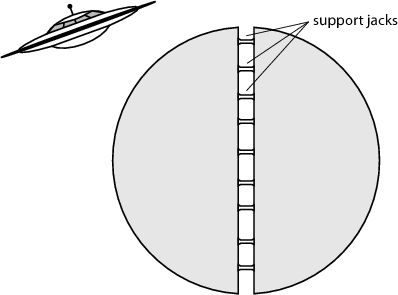Figure 1: The planetoid, cut in half.

(Hint: The equations of the gravitational field are analogous to electrostatics. This problem is equivalent to finding the force needed to keep the two halves of a uniformly charged solid sphere from separating.)

Back to the main page